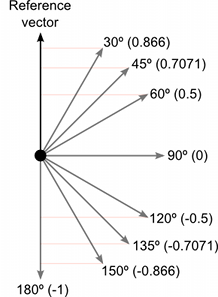# How to determine the direction of an object to the player.

I have a 3rd person game. The thing i want to do is if the object is on my left and right. The rigidbody Constraints lock the Z axis. If the object is on my front and back, The rigidbody constraints lock the X axis.

http://docs.unity3d.com/Manual/UnderstandingVectorArithmetic.html

You can easily use the dot product to know where the object is located

Dot productLet’s define the vector between the player and the object :

``````Vector3 vector = object.transform.position - player.transform.position ;
``````

Then , you have the following :

``````float forwardDotProduct = Vector3.Dot( player.transform.forward, vector ) ;
float rightDotProduct = Vector3.Dot( player.transform.right, vector ) ;

if( forwardDotProduct > 0.70f )
{
// The object is in front of the player
}
else if( forwardDotProduct > 0 )
{
// The object is on the right OR on the left
if( rightDotProduct  > 0 )
// The object is on the right
else
// The object is on the left
}
else
{
// The object is behind the player
}
``````